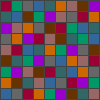Zigzag permutations and quantum operators by Andrew HodgesThis is a brief introduction to the papers: Andrew Hodges and C. V. Sukumar, Bernoulli, Euler, permutations and quantum algebras, Proc. R. Soc. A (2007) 463, 2401-2414 doi:10.1098/rspa.2007.0001 C. V. Sukumar and Andrew Hodges, Quantum algebras and parity-dependent spectra, Proc. R. Soc. A (2007) 463, 2415-2427 doi:10.1098/rspa.2007.0003 Andrew Hodges and C. V. Sukumar are Fellows of Wadham College, University of Oxford.## Secant and tangent numbers

The integer sequences

1, 1, 5, 61, 1385, 50521...

and

1, 2, 16, 272, 7936, 353792...

are well-known in mathematics as the secant number and tangent number sequences respectively.

See Secant number (Wolfram MathWorld), Tangent number (Wolfram MathWorld) and Secant number (Journal of Integer Sequences), Tangent number (Journal of Integer Sequences). The secant numbers are also known as Euler numbers, and the tangent numbers are closely related to the Bernoulli numbers.

We shall refer to the secant numbers as E0, E2, E4... and to the tangent numbers as T1, T3, T5...

Knowing these numbers is equivalent to knowing the Taylor series for the secant and tangent functions respectively. In fact, E2n is just the (2n)th derivative of sec z at z = 0. T2n−1 is just the (2n−1)th derivative of tan z at z = 0.## Zigzag permutations

These sequences have a remarkable combinatorial property. The secant numbers (for even m) and the tangent numbers (for odd m) give the number of alternating or zigzag permutations on m elements.

To see what this means, take the case m=4. Of the 24 permutations of 4 elements, which we can write as 1234, 1243, 1324... 4321, just five have the property of running up-down-up in an alternating fashion. These are the permutations 1423, 1324, 2413, 2314, 3412. This tally agrees with E4=5.

Likewise T5=16 corresponds to the tally of sixteen up-down-up-down alternating permutations on 5 elements, viz. 13254, 14352, 14253, 15243, 15342, 23154, 24153, 24351, 25143, 25341, 34152, 34251, 35142, 35241, 45132, 45231.## A new classification of permutations

All of this is well known, and there are many efficient ways of computing the secant and tangent numbers. Recently, however, Dr C. V. Sukumar and I noticed a rather direct and elementary method for calculating them, based on a simple extension of the concept of alternating permutation.

Consider the two permutations of 8 elements given by 13284657 and 38142756. Both are alternating (up-down-up-down-up-down-up), but they differ in where the 'ups' and 'downs' occur. In 13284657, the 4 is at a 'low' point between two greater numbers, whilst in 38142756 the 4 is at a 'high' point between two smaller numbers.

We label 'low' points by R, and 'high' points by L. The first and last numbers in the sequence can be brought into the scheme as shown in the diagrams. Then the fact that the zigzag begins with an 'up' and ends with a 'up' is coded into the fact that the first number is an R and the last number an L.

The classification is specified more formally in our first paper, but these zigzag pictures convey the idea in a natural way.

We shall say that 13284657 has secant zigzag type RRLRRLLL, meaning that 1 is a low, 2 is a low, 3 is a high, 4 is a low, 5 is a low, and 6, 7, and 8 are highs. Similarly, 38142756 has secant zigzag type RRRLRLLL.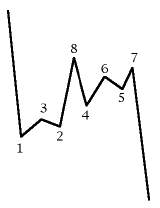13284657
as a secant zigzag path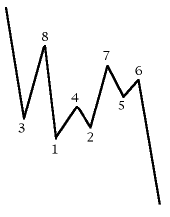38142756
as a secant zigzag path

To illustrate the kind of structure that emerges, we consider the classification for alternating permutations on 8 elements. The norm sign indicates the size of the classes.

• |RRRRLLLL| = 576
• |RRRLRLLL| = 324
• |RRRLLRLL| = |RRLRRLLL| = 144
• |RRLRLRLL| = 64
• |RRRLLLRL| = |RLRRRLLL| = 36
• |RRLRLLRL| = |RLRRLRLL| = 16
• |RRLLRRLL| = 16
• |RRLLRLRL| = |RLRLRRLL| = 4
• |RLRRLLRL| = 4
• |RLRLRLRL| = 1
This last class contains just the permutation 21436587. The class sizes sum to E8=1385.

The reason for the choice of R and L is that the non-empty classes correspond exactly to the paths on the non-negative integers starting and ending at 0 and moving Right or Left by one step. Thus the class RRLRRLLL corresponds to the path (0,1,2,1,2,3,2,1,0) and RRRLRLLL to the path (0,1,2,3,2,3,2,1,0). The number of such paths is well known to be the Catalan Number which in this case is 8!/(4! × 5!) = 14.

This classification has been chosen in just such a way that the every alternating permutation is characterised by a sequence of R's and L's. But it can be extended to all permutations by writing an S when a position is neither R nor L. For instance, the permutation 58372461 is of type SRRSRLLL. These classes can also be mapped into paths on the non-negative integers, by allowing S steps which are stationary, e.g. SRRSRLLL corresponds to the path (0,0,1,2,2,3,2,1,0).

We could apply this classification to permutations of an odd number of elements, but it would not pick out the alternating permutations correctly. To do this, we need to define a different classification, the tangent zigzag classes. Considering permutations on 7 elements, the alternating permutations run up-down-up-down-up-down, and this is naturally accounted for by writing R' for low points, L' for high points, S' for points which are neither high nor low, and dealing with the end points as in the illustrated example. Since 1 is always a low point in this classification, in any permutation, its R' is omitted as redundant. Thus the alternating permutation 4713265 is of type R'L'R'R'L'L' (as 2, 4, and 5 are low points; 3, 6, and 7 are high points).

Both the secant and the tangent classes can now be written down for permutations of both even and odd degree, but the secant classes serve to pick out the alternating permutations of even degree, and the tangent classes pick out alternating permutations of odd degree.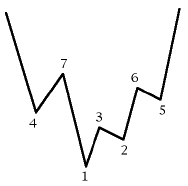4713265
as a tangent zigzag path## An operator calculus for permutations

How can the sizes of these classes be calculated efficiently? It is now very helpful to extend the definitions. If X and Y are zigzag classes, with sizes |X| and |Y|, define |X + Y| to be |X| + |Y| and more generally |αX + βY| = α|X| + β|Y|. To see the value of this extension, consider the case of E4 = 5. Using the classification we have developed, we have

E4 = |RRLL| + |RLRL| = 4 + 1 = 5

since these are the only non-empty secant zigzag classes. But by adding on fourteen further zero terms we also have

E4 = |RRRR| + |RRRL| + |RRLR| + |RRLL| + ... + |LLLL|

= |(R + L)4|.

Likewise in general, we have the elegant formulas:

E2n = |(R + L)2n|

T2n+1 = |(R' + L')2n|.

We also have:

|(R + L + S)2n| = (2n)!

|(R' + L' + S')2n| = (2n+1)!

The next observation is that with this extension, our R, L, S etc. can be treated as operators on a vector space. They are non-commuting operators, because RL is not the same as LR. In fact we can define the commutator [R, L] = RL − LR. This allows us to express our extraordinarily simple main result as follows:

### Main Theorem:

The commutators of R, L and S are given by:

[R, L] = S,   [R, S] = 2R,   [S, L] = 2L

Also

[R', L'] = S',   [R', S'] = 2R',   [S', L'] = 2L'

The proofs of these statements require only simple counting arguments and are given in our first paper. These, together with some other simple facts, imply that for any X (a sequence of R, L and S operators):

For p ≥ 0, |RpSX| = (2p + 1) |RpX|

For p ≥ 0, |RpLX| = (p + 1)2 |Rp−1X|

and similarly

For p ≥ 0, |R'pS'X'| = 2(p + 1) |R'pX'|

For p ≥ 0, |R'pL'X'| = (p + 1)(p + 2) |R'p−1X'|

from which the size of any given class can be evaluated recursively.

For evaluation of the secant and tangent numbers it is not necessary to work out the sizes of the individual classes but instead to evaluate |(R + L)2n|, |(R' + L')2n| directly. The results can be presented in a form which slightly generalises the idea of the Pascal triangle: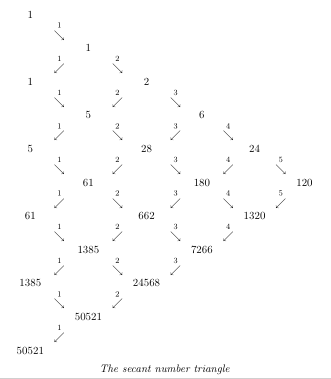These triangles can be expressed as very simple recursive algorithms. They are already well-known but our analysis gives them a new interpretation — both in terms of permutations and in terms of Hilbert space structure.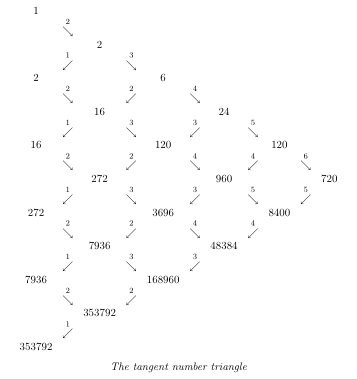A similar recursive triangle for the tangent numbers.## Deeper structure and quantum mechanical connections

The commutation relations for R, L, and S are well-known in quantum mechanics. They arise when we have an annihilation operator a, and its adjoint creation operator a*, with the commutator [a, a*] = 1. Then

r = a2,   l = a*2   and   s = 1/2(aa* + a*a)

satisfy the same commutation relations as do the R, L and S. Does this mean there are analogous annihilation and creation operators for the permutations, which behave like square roots of the R and L operators? Almost, but not quite.

We show in the papers that it is possible and useful to define A and A* operators which are more primitive than the R, L, S, R', L', S', and which bring them all into a single scheme. However, these A and A* do not satisfy the standard commutation relations for annnihilation and creation operators, but a generalisation of them with a parity-dependent term. In our second paper we analyse these commutation relations in greater generality.

It seems to us that there may be further surprising results about permutations and operator structures to be found from this starting-point. A particularly interesting feature is that the duality between secant and tangent numbers can be expressed in terms of an anti-commuting operator.

One question we have explored is that of the parallel structure for transpositions, i.e. those permutations which simply swap elements in pairs. As an example, the permutation 53271846 is a transposition, swapping the pairs (15)(23)(47)(68). First we define a new classification for transpositions, in which a number is marked C if its partner is greater, and D if its partner is smaller. Thus this transposition is of type CCDCDCDD. In this case it turns out that C and D behave as annihilation and creation operators which satisfy the standard commutation relation [C, D] = 1.

The analogues of the alternating permutations are then those transpositions which are generated entirely by C2 and D2. (For instance (15)(27)(38)(46) is of type CCCCCDDDD.) Computing the sizes of these classes is equivalent to calculating certain amplitudes which arise in quantum optics.

In our second paper we compute the total number of 'alternating transpositions', as so defined, by a purely combinatorial argument, finding agreement with the standard answer from quantum mechanics. The series runs 1, 2, 28, 1112, 87568, 11447072... and has generating function √(sec 2z).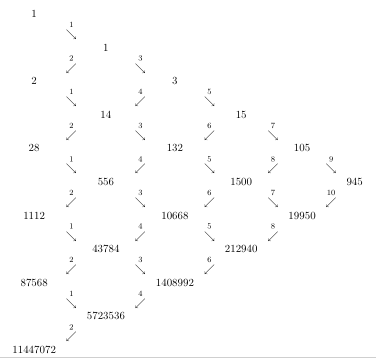Recursive triangle for calculating the number of alternating transpositions.This is a brief introduction to the papers: Andrew Hodges and C. V. Sukumar, Bernoulli, Euler, permutations and quantum algebras, Proc. R. Soc. A (2007) 463, 2401-2414 doi:10.1098/rspa.2007.0001 C. V. Sukumar and Andrew Hodges, Quantum algebras and parity-dependent spectra, Proc. R. Soc. A (2007) 463, 2415-2427 doi:10.1098/rspa.2007.0003 Andrew Hodges and C. V. Sukumar are Fellows of Wadham College, University of Oxford.More Cool MathsAlan Turing Home Page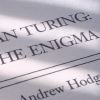Alan Turing: the enigma